Algebra 2 6-5 Complete Lesson: Solving Square Root and Other Radical Equations
starstarstarstarstarstarstarstarstarstar
4.5 (1 rating)
by Matthew Richardson
| 24 Questions
Note from the author:
A complete formative lesson with embedded slideshow, mini lecture screencasts, checks for understanding, practice items, mixed review, and reflection. I create these assignments to supplement each lesson of Pearson's Common Core Edition Algebra 1, Algebra 2, and Geometry courses. See also mathquest.net and twitter.com/mathquestEDU.The outlined content above was added from outside of Formative.
1
10 pts
Solve It! You are a passenger in the car. You are using a cell phone that connects with the cell phone tower shown. The tower has an effective range of 6 mi. How many miles do you have to finish your call?

You have about 5.2 mi to finish your call.
You have about 25.7 mi to finish your call.
You have 3 mi to finish your call.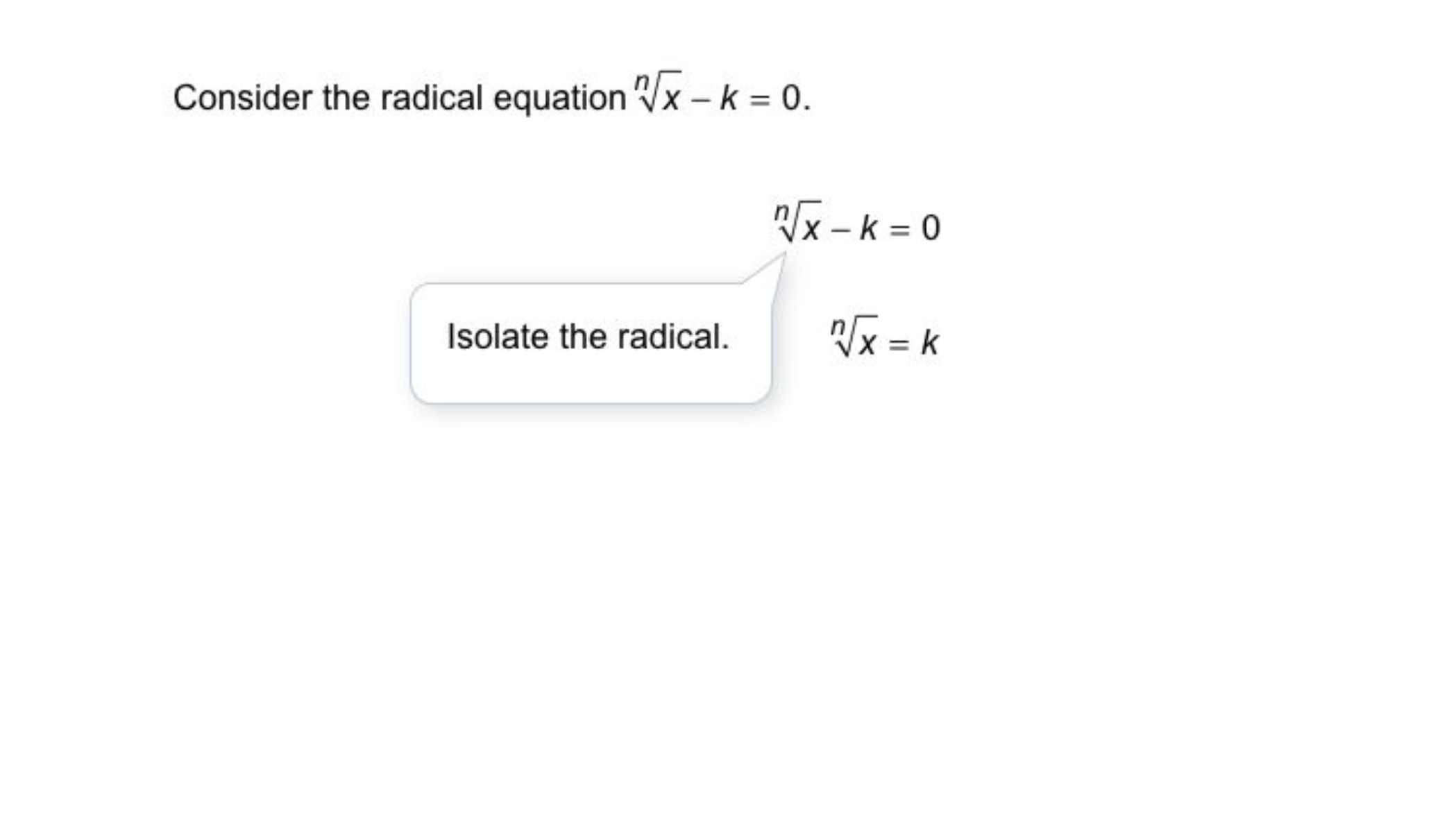2
2
10 pts
Problem 1 Got It?
A
B
C
D3
3
10 pts
Problem 2 Got It?
A
B
C
D4
4
10 pts
Problem 3 Got It?
A
B
C
D5
10 pts
Problem 4 Got It? Solve the radical equation. Check your results.

x = 10
x = 0, x = 1
x = 1, x = 10
x = 1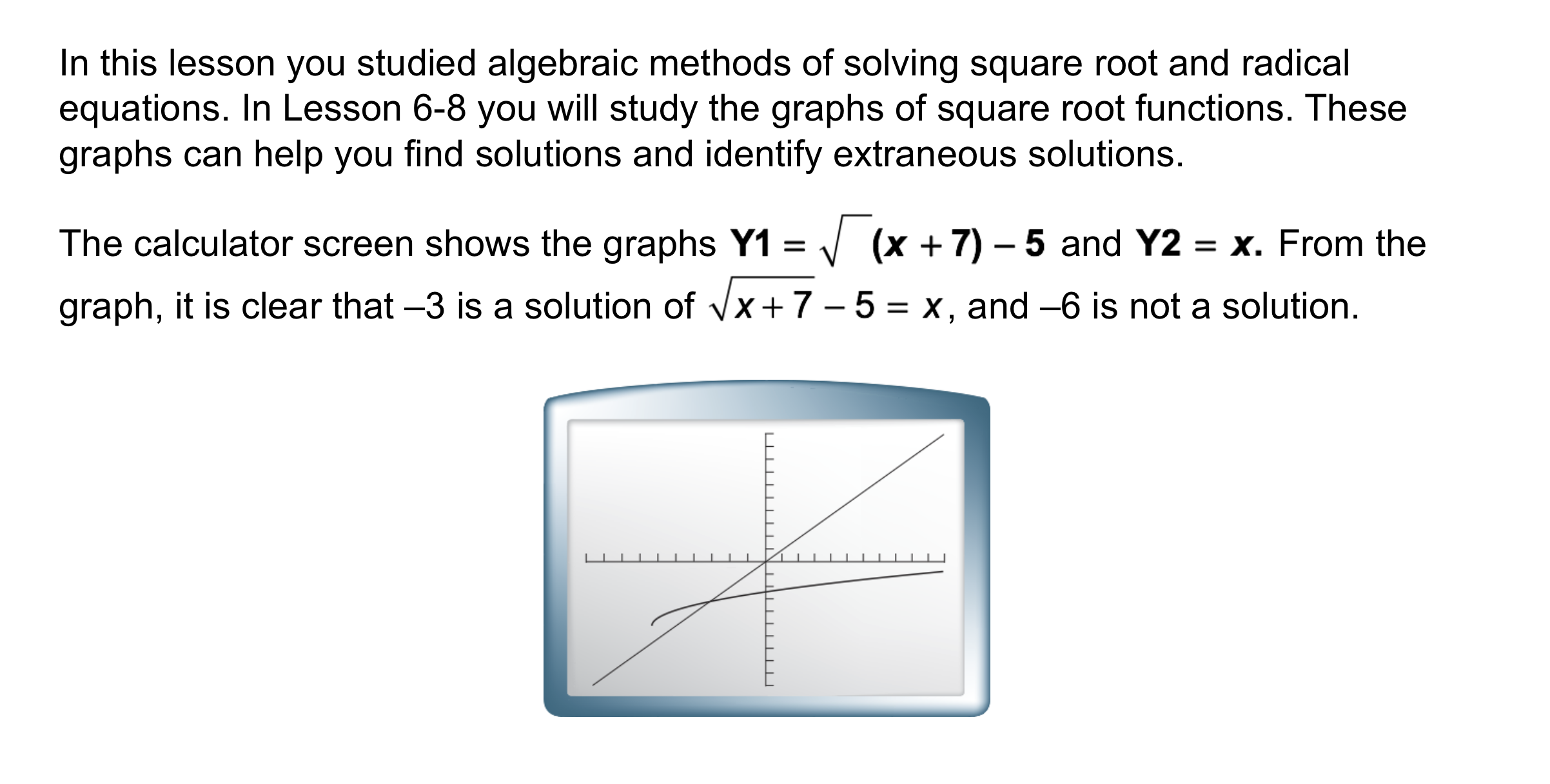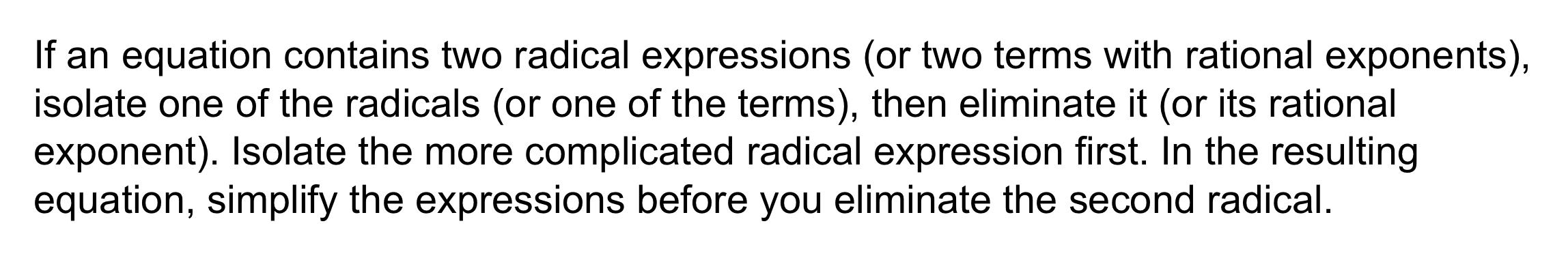7
7
10 pts
Problem 5 Got It?
A
B
C
D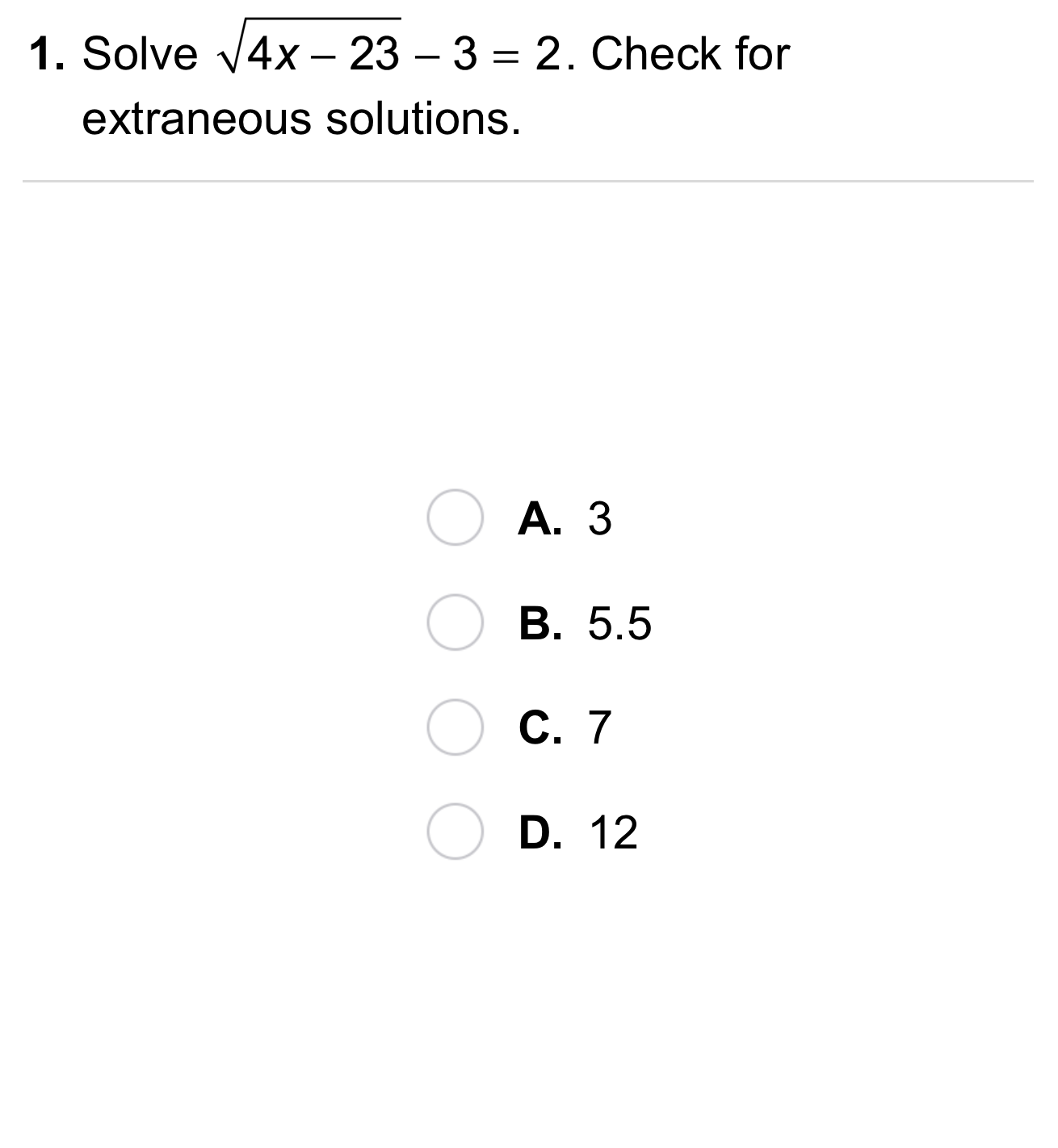8
8
10 pts
A
B
C
D9
9
10 pts
A
B
C
D10
10
10 pts
A
B
C
D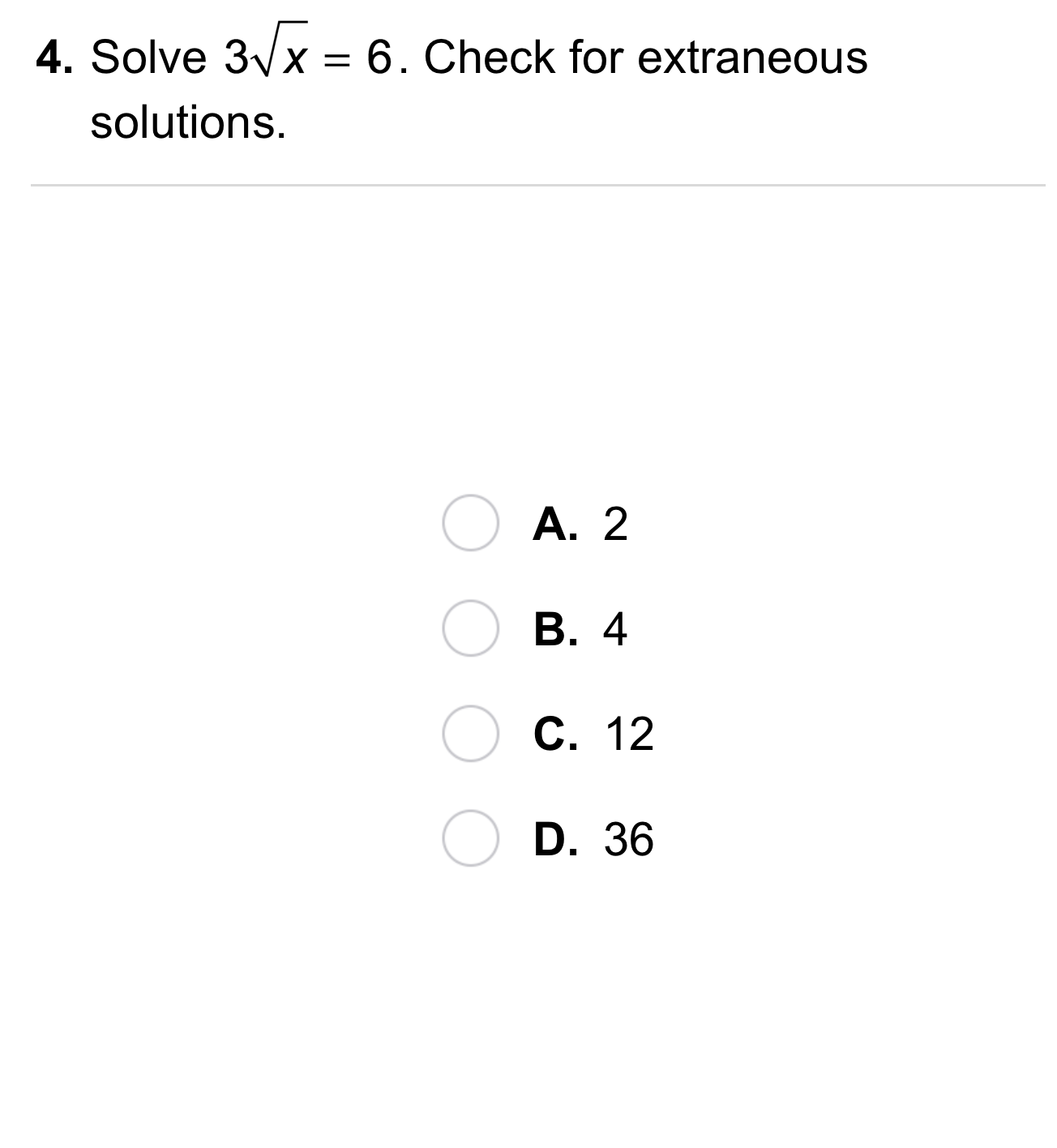11
11
10 pts
A
B
C
D12
12
10 pts
A
B
C
D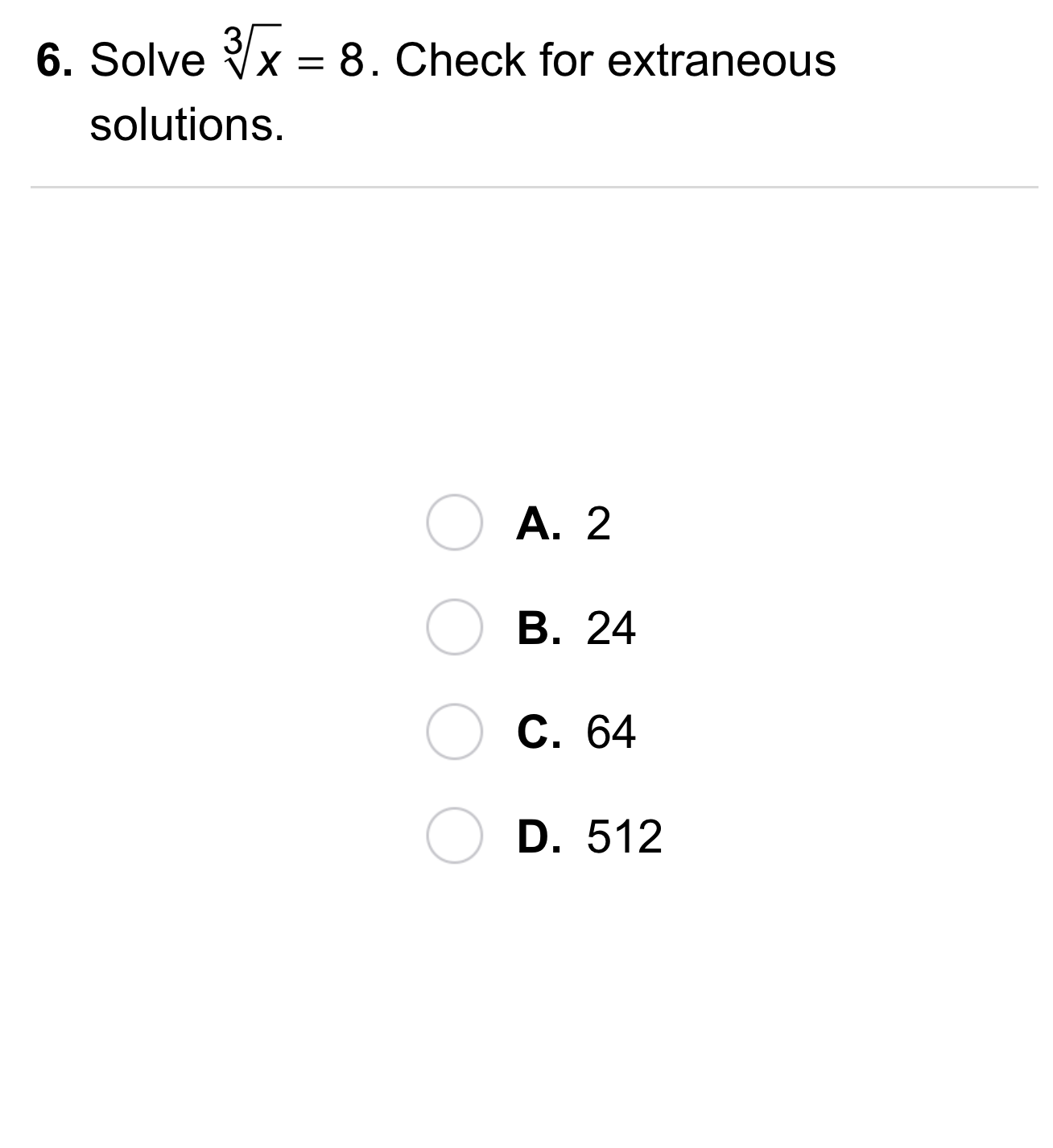13
13
10 pts
A
B
C
D14
10 pts
Vocabulary: Which value, 12 or 3, is an extraneous solution of the equation?
Enter only a number.
15
10 pts
Compare and Contrast: How is solving a square root equation similar to solving an absolute value equation? How is it different?16
10 pts
Review Lesson 6-4: Match each number on the left with its simplified form on the right.

• 3
• 2
• 125
17
10 pts
Review Lesson 4-5: Consider the quadratic equation below.
1. Drag the factors of the quadratic expression from the left column into the top category on the right.
2. Drag the solution(s) of the quadratic equation from the left column into the bottom category on the right.
• x
• (3x + 2)
• (3x + 1)
• (x + 2)

• Factors of the expression:

• Solution(s) of the equation:

18
10 pts
Review Lesson 2-1: Categorize values from the left to identify the domain and range of the relation. Then drag either Yes or No to respond to the question.

• 0
• 1
• 2
• Yes
• No
• Domain:
• Range:
• Is the relation a function?19
10 pts
Vocabulary Review: Which equation is equivalent to the equation below?

20
10 pts
Vocabulary Review: Match each solution from the left with the equation it solves on the right.
• -10
• 10
• 2
• -2

21
10 pts
Vocabulary Review: What is the radicand in the expression?22
10 pts
Use Your Vocabulary: Categorize each statement as true or false.
• The reciprocal of -1 is itself.
• A decimal has no reciprocal.
• The reciprocal of a negative number is negative.
• The only real number without a reciprocal is 0.
• True
• False
23
100 pts
Notes: Take a clear picture or screenshot of your Cornell notes for this lesson. Upload it to the canvas. Zoom and pan as needed.

For a refresher on the Cornell note-taking system, click here.
24
10 pts
Reflection: Math Success
Add to my formatives list

Formative uses cookies to allow us to better understand how the site is used. By continuing to use this site, you consent to the Terms of Service and Privacy Policy.